This is “Solving Systems of Linear Inequalities (Two Variables)”, section 4.5 from the book Beginning Algebra (v. 1.0). For details on it (including licensing), click here.

Has this book helped you? Consider passing it on:
Creative Commons supports free culture from music to education. Their licenses helped make this book available to you.
DonorsChoose.org helps people like you help teachers fund their classroom projects, from art supplies to books to calculators.

## 4.5 Solving Systems of Linear Inequalities (Two Variables)

### Learning Objectives

1. Check solutions to systems of linear inequalities with two variables.
2. Solve systems of linear inequalities.

## Solutions to Systems of Linear Inequalities

A system of linear inequalitiesA set of two or more linear inequalities that define the conditions to be considered simultaneously. consists of a set of two or more linear inequalities with the same variables. The inequalities define the conditions that are to be considered simultaneously. For example,We know that each inequality in the set contains infinitely many ordered pair solutions defined by a region in a rectangular coordinate plane. When considering two of these inequalities together, the intersection of these sets defines the set of simultaneous ordered pair solutions. When we graph each of the above inequalities separately, we have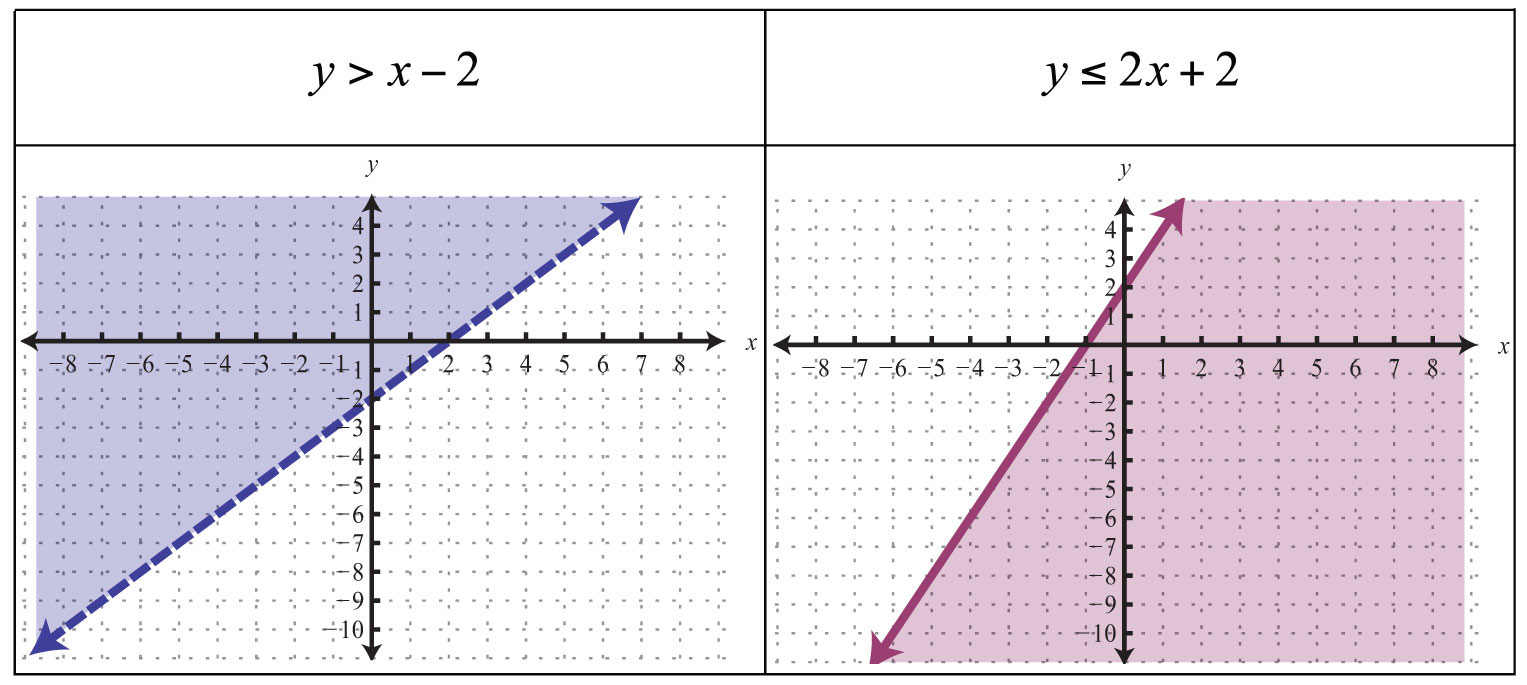When graphed on the same set of axes, the intersection can be determined.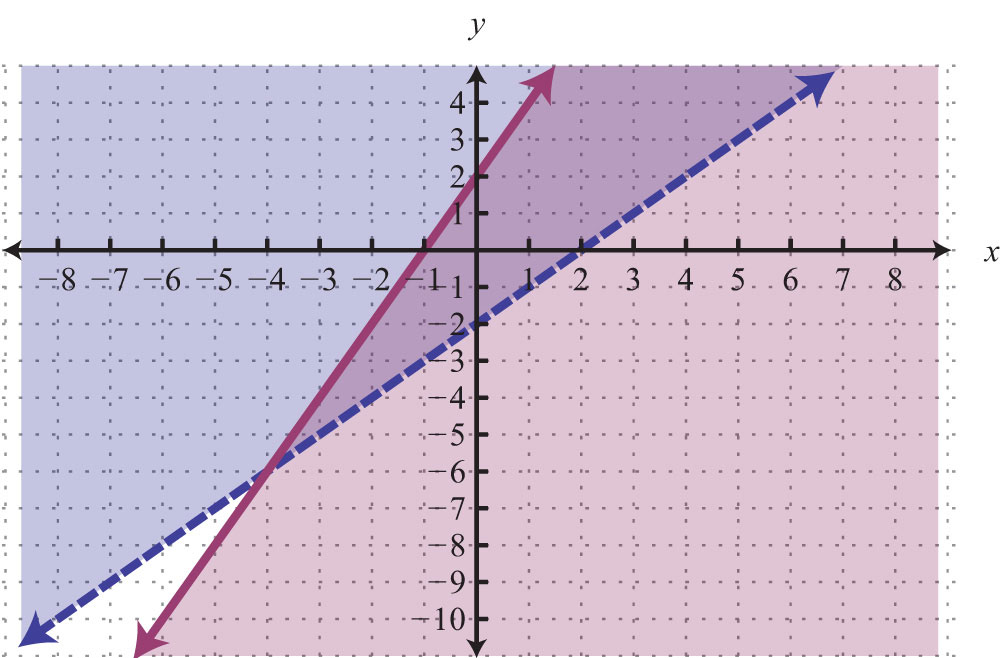The intersection is shaded darker and the final graph of the solution set is presented as follows: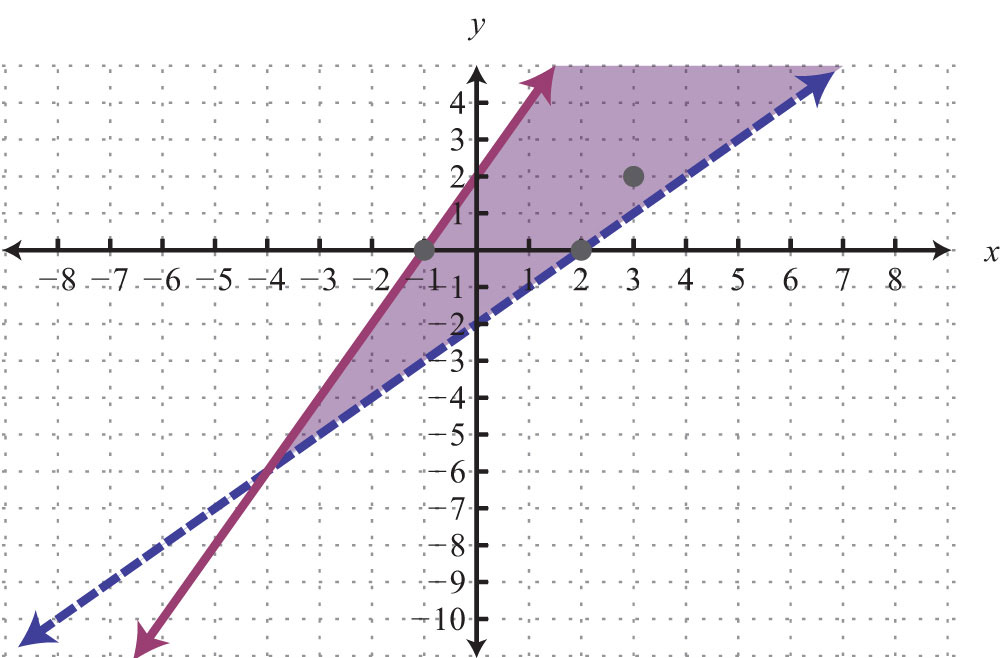The graph suggests that (3, 2) is a solution because it is in the intersection. To verify this, show that it solves both of the original inequalities: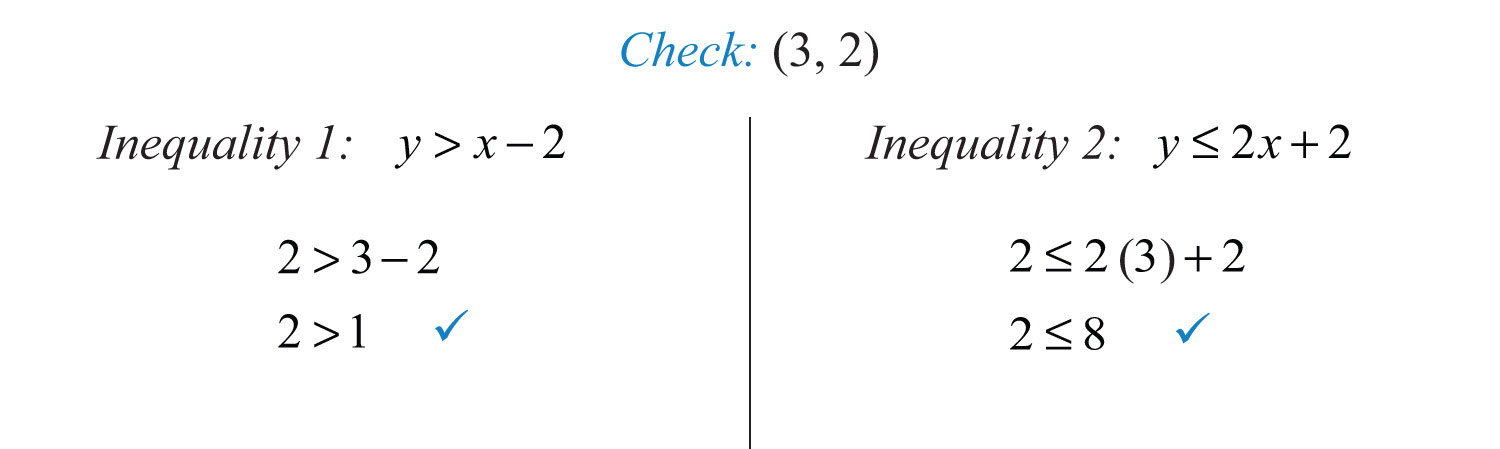Points on the solid boundary are included in the set of simultaneous solutions and points on the dashed boundary are not. Consider the point (−1, 0) on the solid boundary defined by $y=2x+2$ and verify that it solves the original system: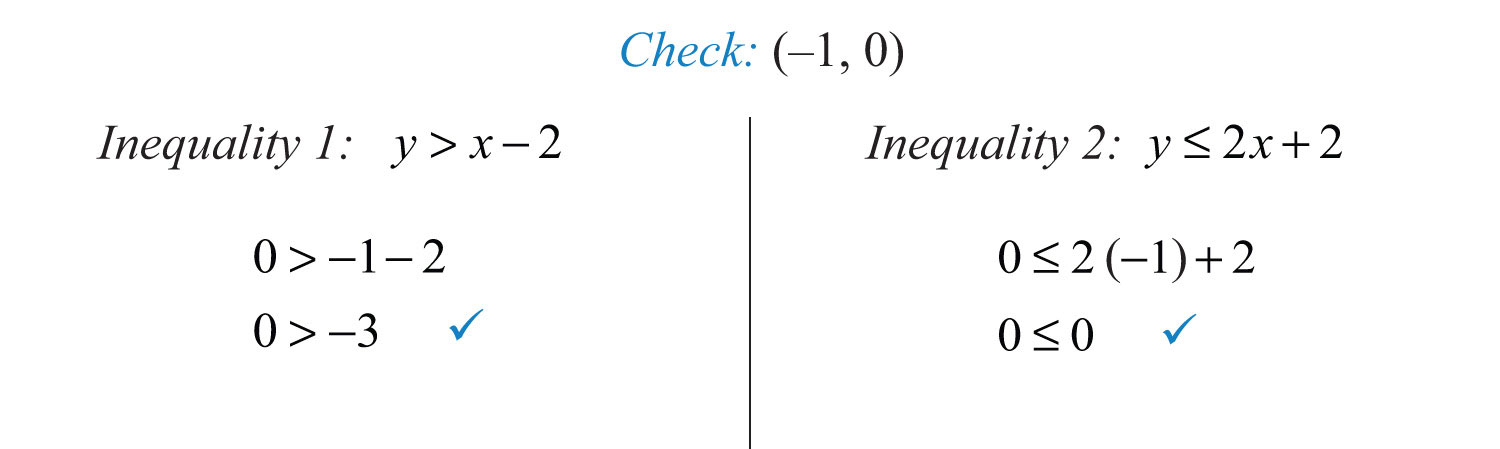Notice that this point satisfies both inequalities and thus is included in the solution set. Now consider the point (2, 0) on the dashed boundary defined by $y=x−2$ and verify that it does not solve the original system: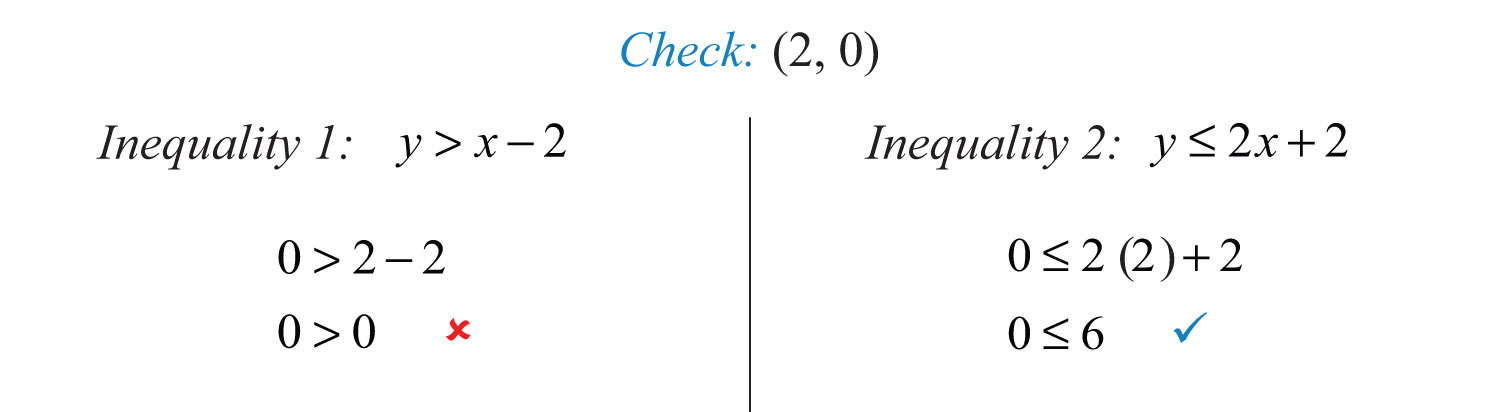This point does not satisfy both inequalities and thus is not included in the solution set.

## Solving Systems of Linear Inequalities

Solutions to a system of linear inequalities are the ordered pairs that solve all the inequalities in the system. Therefore, to solve these systems, graph the solution sets of the inequalities on the same set of axes and determine where they intersect. This intersection, or overlap, defines the region of common ordered pair solutions.

Example 1: Graph the solution set: ${−2x+y>−43x−6y≥6$.

Solution: To facilitate the graphing process, we first solve for y.For the first inequality, we use a dashed boundary defined by $y=2x−4$ and shade all points above the line. For the second inequality, we use a solid boundary defined by $y=12x−1$ and shade all points below. The intersection is darkened.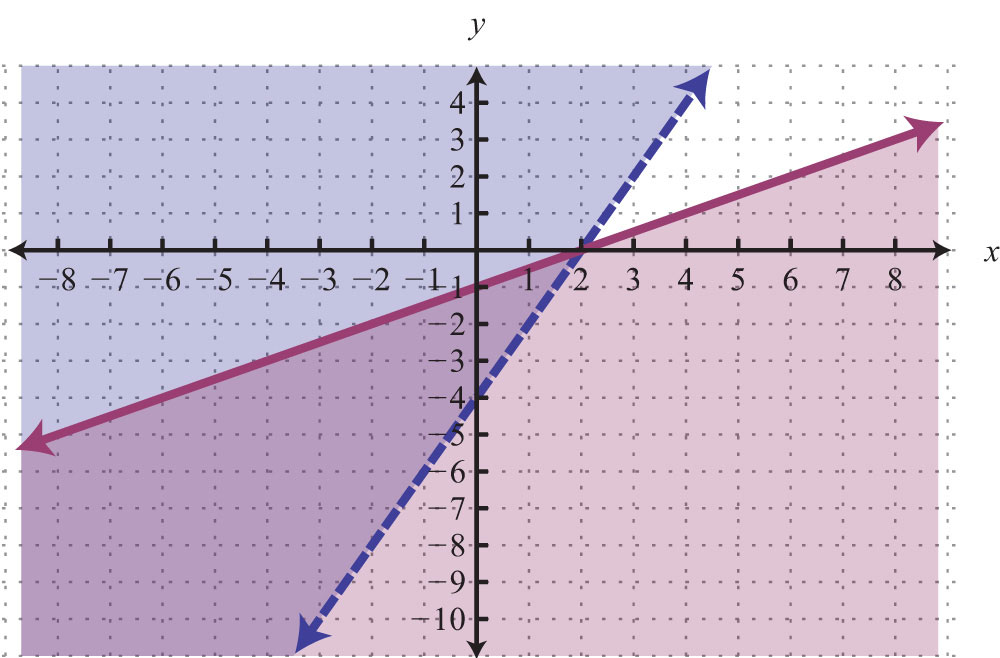Now we present the solution with only the intersection shaded.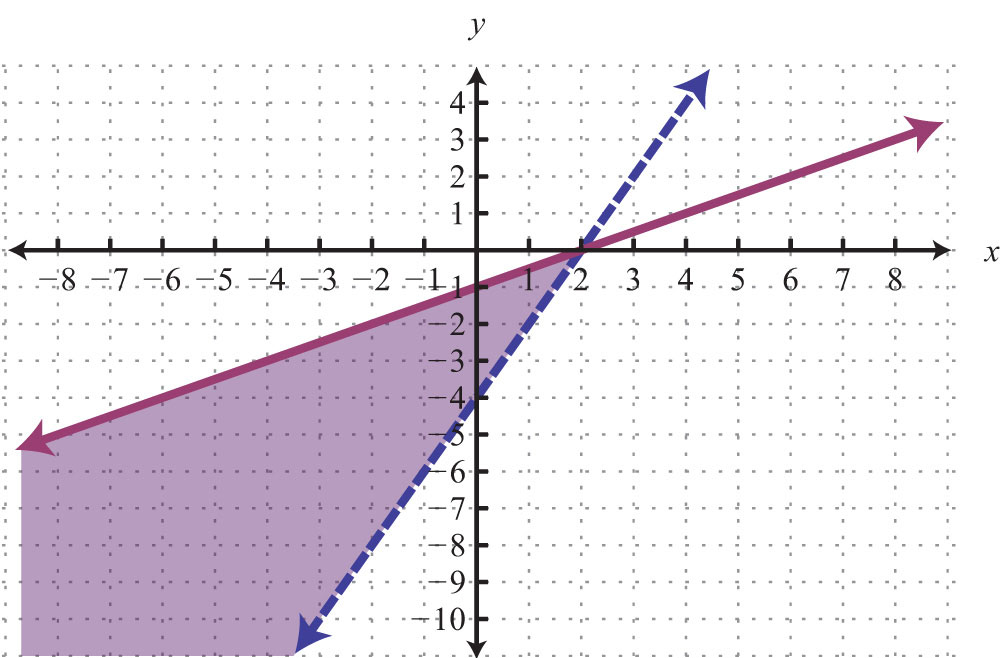Example 2: Graph the solution set: ${−2x+3y>64x−6y>12$.

Solution: Begin by solving both inequalities for y.Use a dashed line for each boundary. For the first inequality, shade all points above the boundary. For the second inequality, shade all points below the boundary.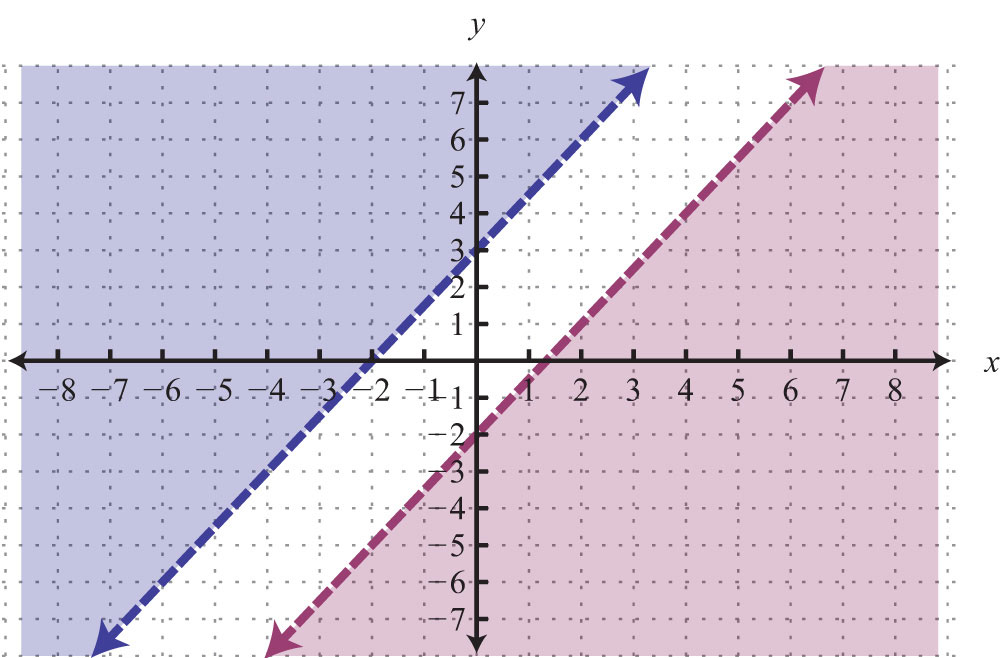As you can see, there is no intersection of these two shaded regions. Therefore, there are no simultaneous solutions.

Answer: No solution, $∅$

Example 3: Graph the solution set: ${y≥−4y.

Solution: The intersection of all the shaded regions forms the triangular region as pictured darkened below: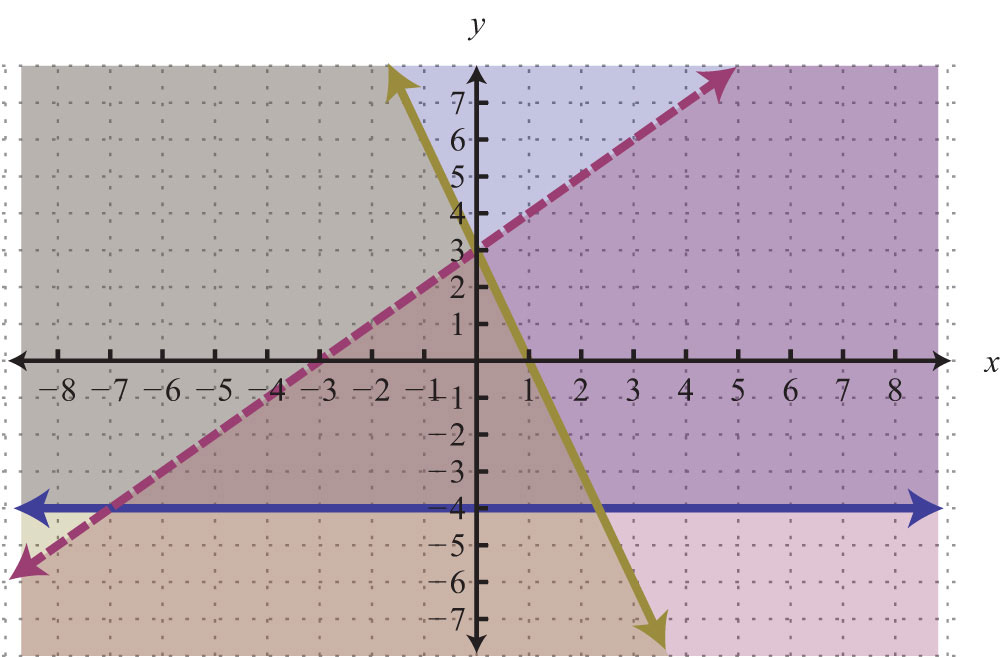After graphing all three inequalities on the same set of axes, we determine that the intersection lies in the triangular region pictured.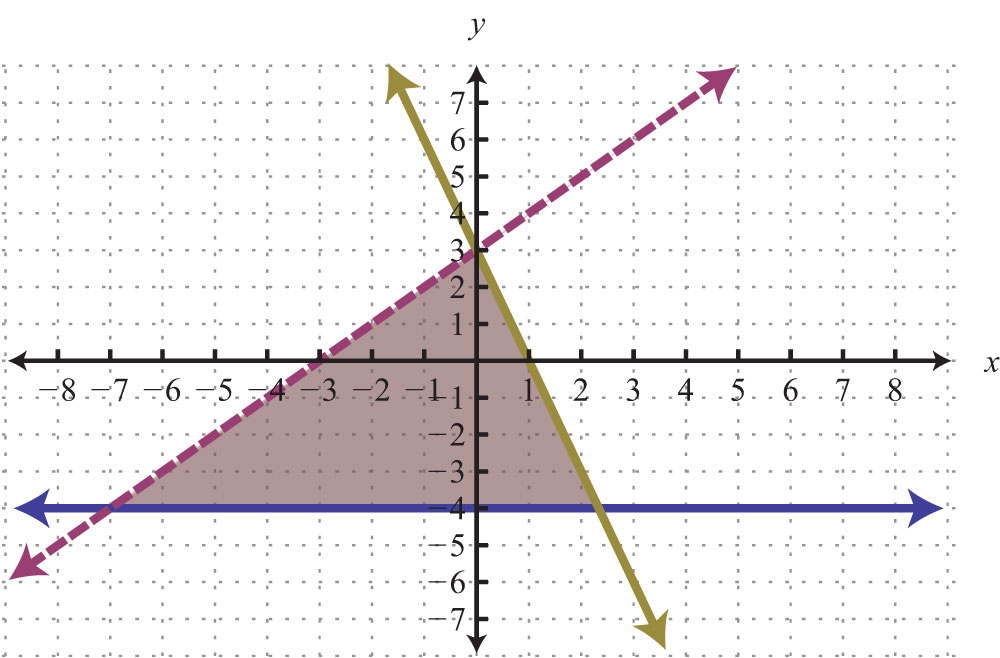The graphic suggests that (−1, 1) is a common point. As a check, substitute that point into the inequalities and verify that it solves all three conditions.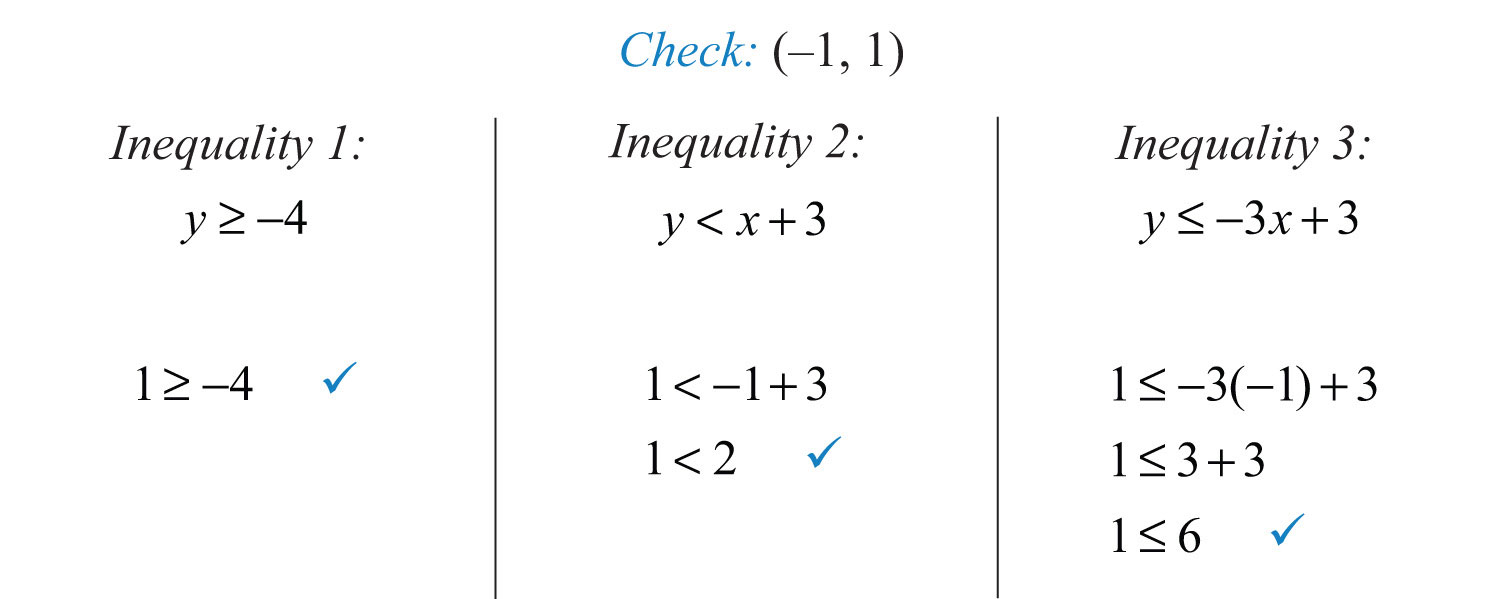### Key Takeaway

• To solve systems of linear inequalities, graph the solution sets of each inequality on the same set of axes and determine where they intersect.

### Topic Exercises

Part A: Solving Systems of Linear Inequalities

Determine whether the given point is a solution to the given system of linear equations.

1. (3, 2); ${y≤x+3y≥−x+3$

2. (−3, −2); ${y<−3x+4y≥2x−1$

3. (5, 0); ${y>−x+5y≤34x−2$

4. (0, 1); ${y<23x+1y≥52x−2$

5. $(−1, 83)$; ${−4x+3y≥−122x+3y<6$

6. (−1, −2); ${−x+y<0x+y<0x+y<−2$

Part B: Solving Systems of Linear Inequalities

Graph the solution set.

7. ${y≤x+3y≥−x+3$

8. ${y<−3x+4y≥2x−1$

9. ${y>xy<−1$

10. ${y<23x+1y≥52x−2$

11. ${y>−x+5y≤34x−2$

12. ${y>35x+3y<35x−3$

13. ${x+4y<12−3x+12y≥−12$

14. ${−x+y≤62x+y≥1$

15. ${−2x+3y>34x−3y<15$

16. ${−4x+3y≥−122x+3y<6$

17. ${5x+y≤4−4x+3y<−6$

18. ${3x+5y<15−x+2y≤0$

19. ${x≥05x+y>5$

20. ${x≥−2y≥1$

21. ${x−3<0y+2≥0$

22. ${5y≥2x+5−2x<−5y−5$

23. ${x−y≥0−x+y<1$

24. ${−x+y≥0y−x<1$

25. ${x>−2x≤2$

26. ${y>−1y<2$

27. ${−x+2y>83x−6y≥18$

28. ${−3x+4y≤46x−8y>−8$

29. ${2x+y<3−x≤12y$

30. ${2x+6y≤6−13x−y≤3$

31. ${y<3y>xx>−4$

32. ${y<1y≥x−1y<−3x+3$

33. ${−4x+3y>−12y≥22x+3y>6$

34. ${−x+y<0x+y≤0x+y>−2$

35. ${x+y<2x<3−x+y≤2$

36. ${y+4≥012x+13y≤1−12x+13y≤1$

37. Construct a system of linear inequalities that describes all points in the first quadrant.

38. Construct a system of linear inequalities that describes all points in the second quadrant.

39. Construct a system of linear inequalities that describes all points in the third quadrant.

40. Construct a system of linear inequalities that describes all points in the fourth quadrant.

1: Yes

3: No

5: No

7: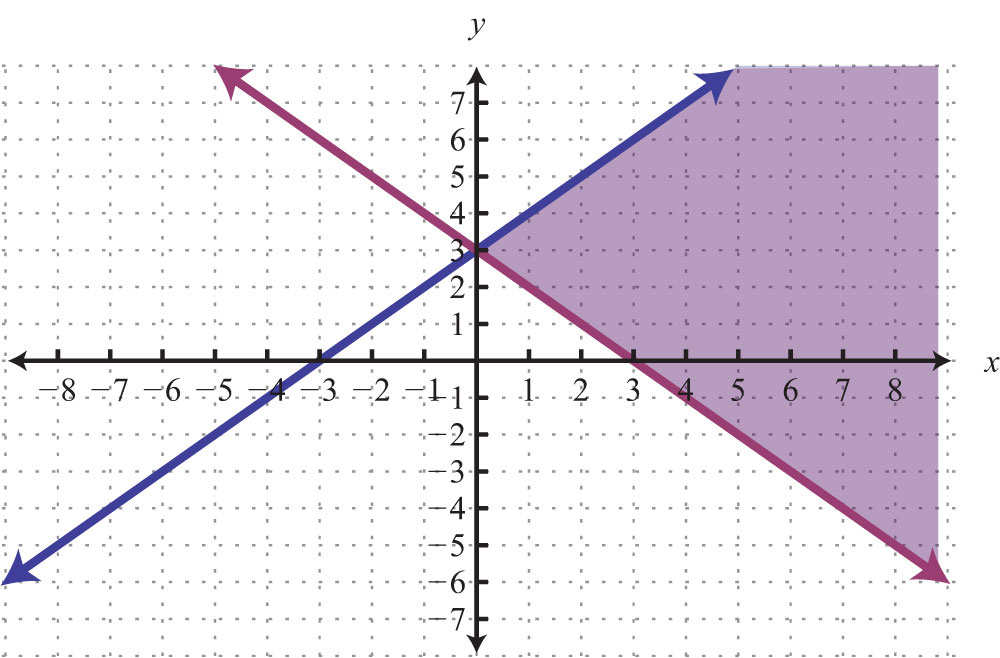9: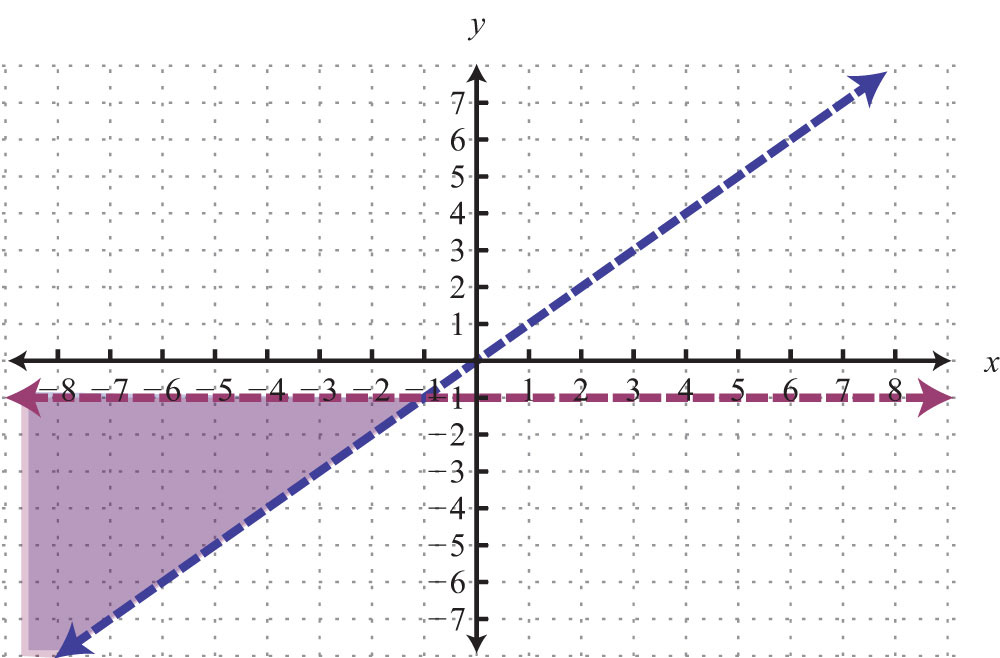11: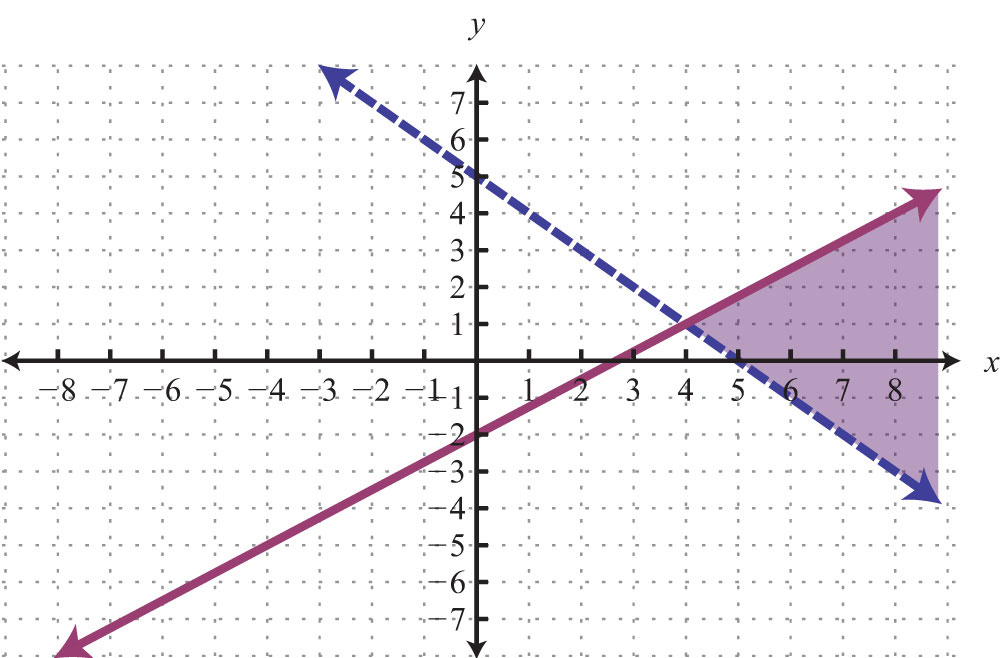13: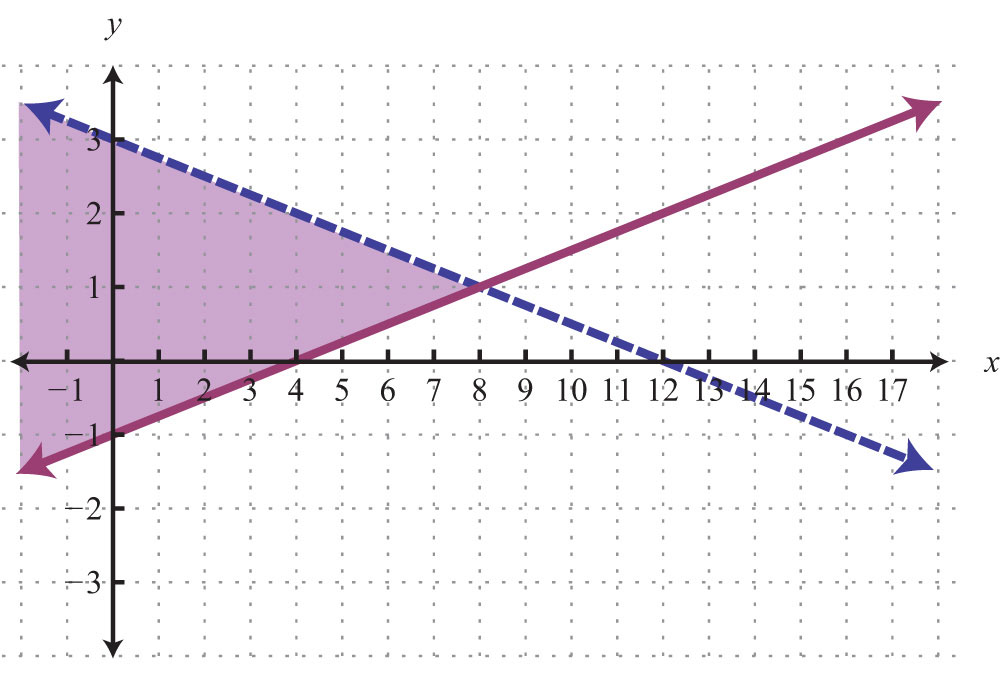15: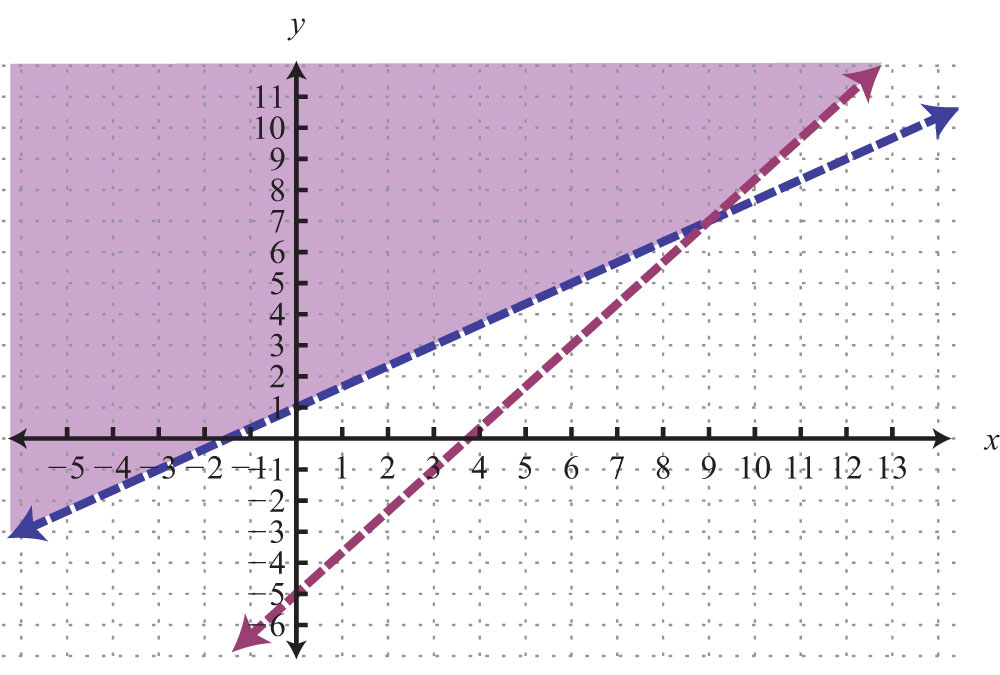17: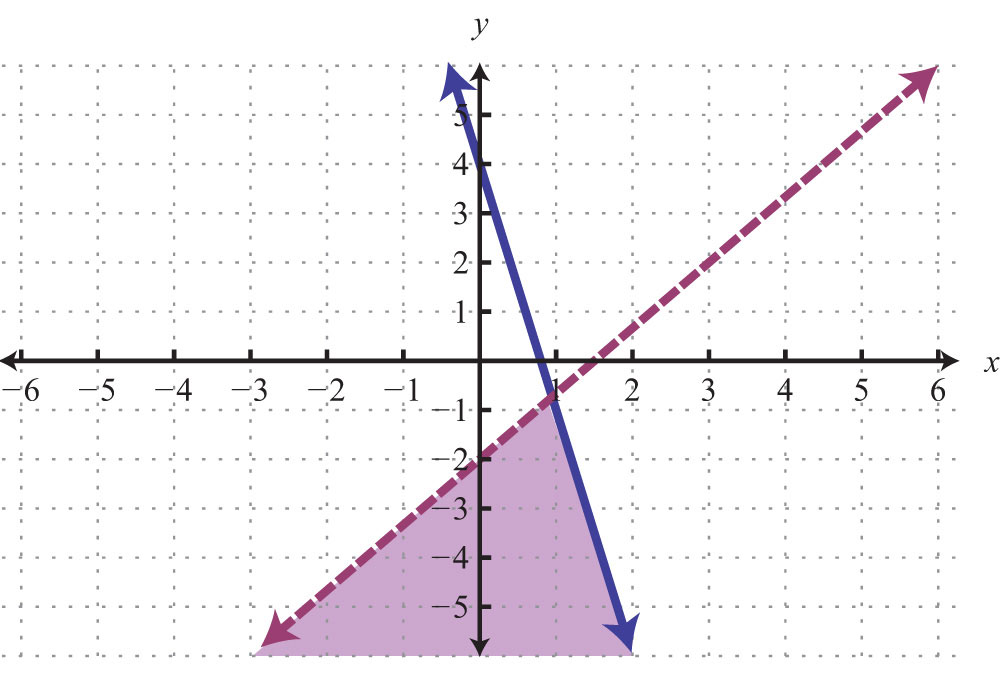19: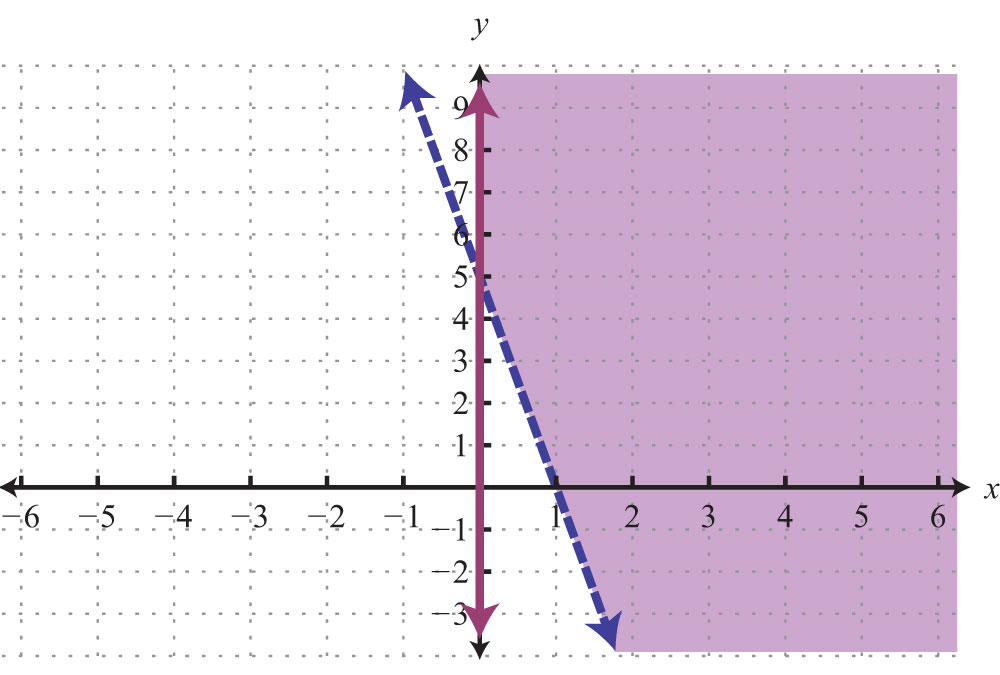21: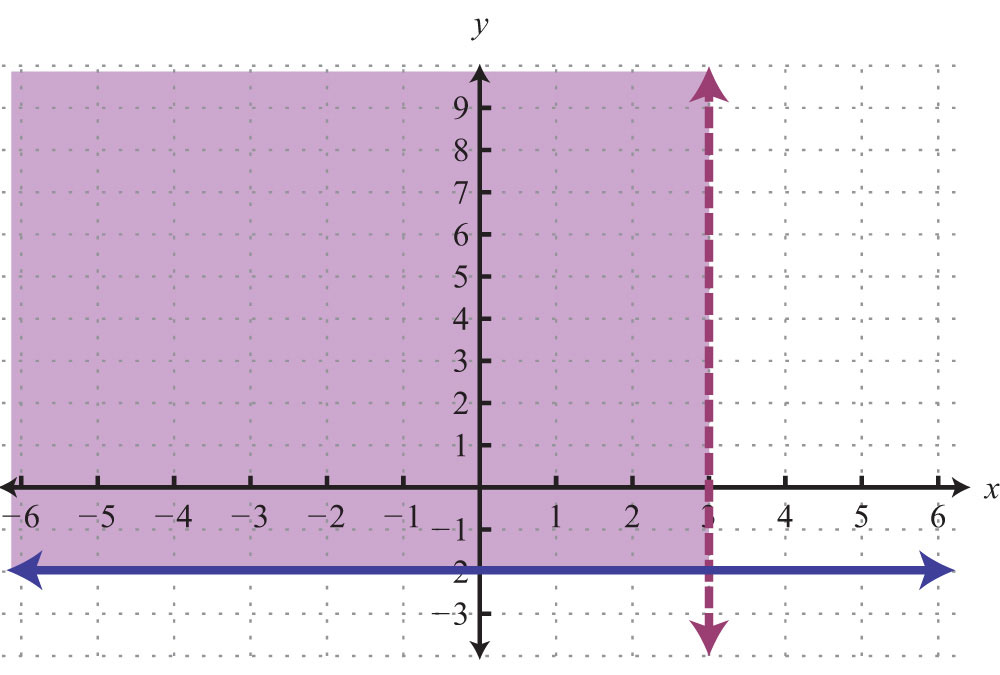23: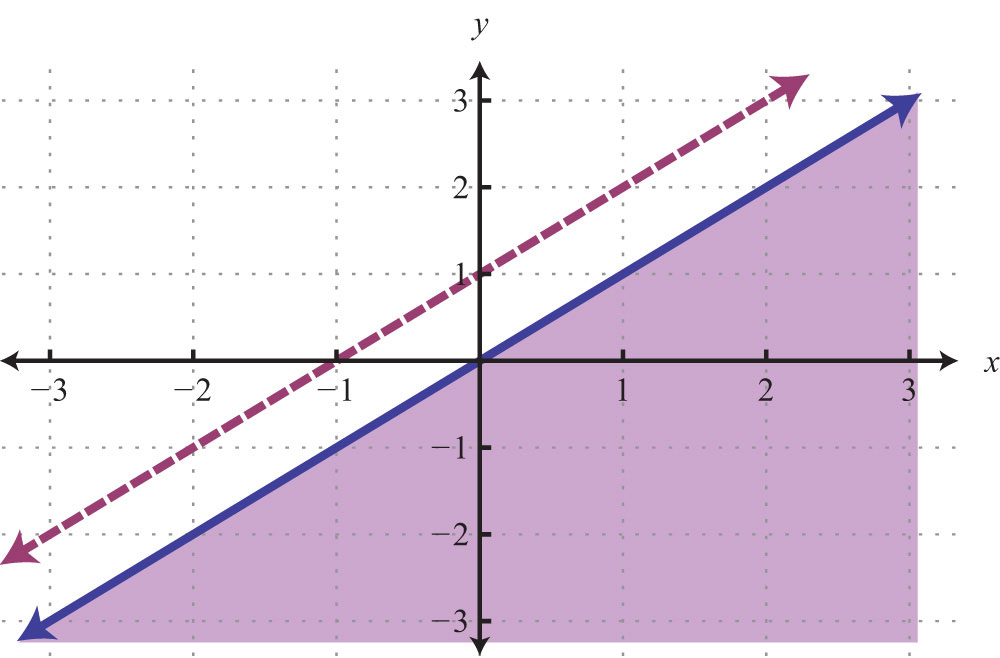25: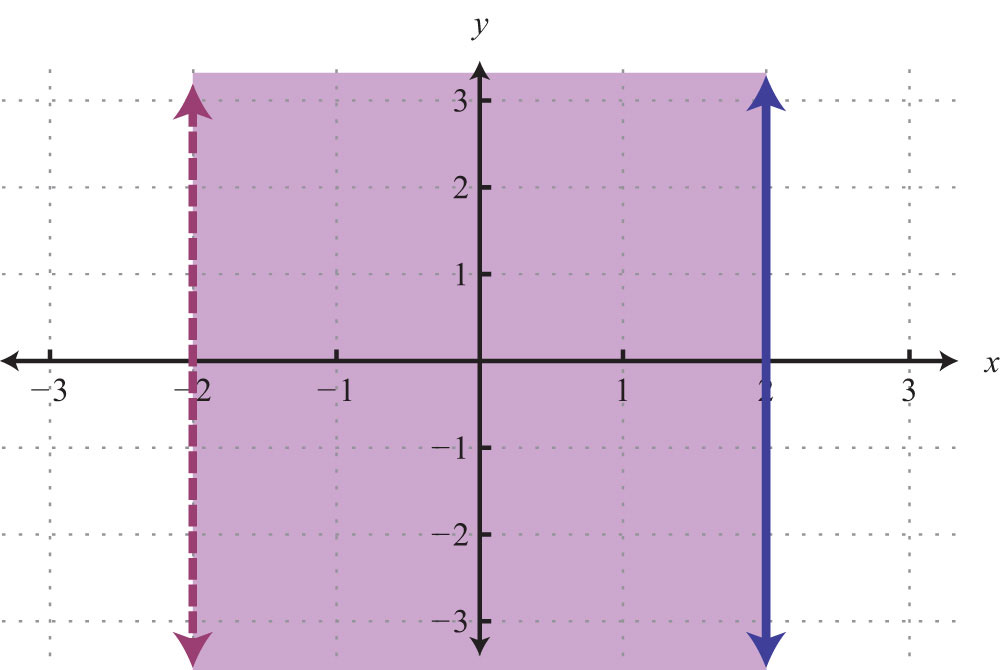27: No solution, $∅$

29: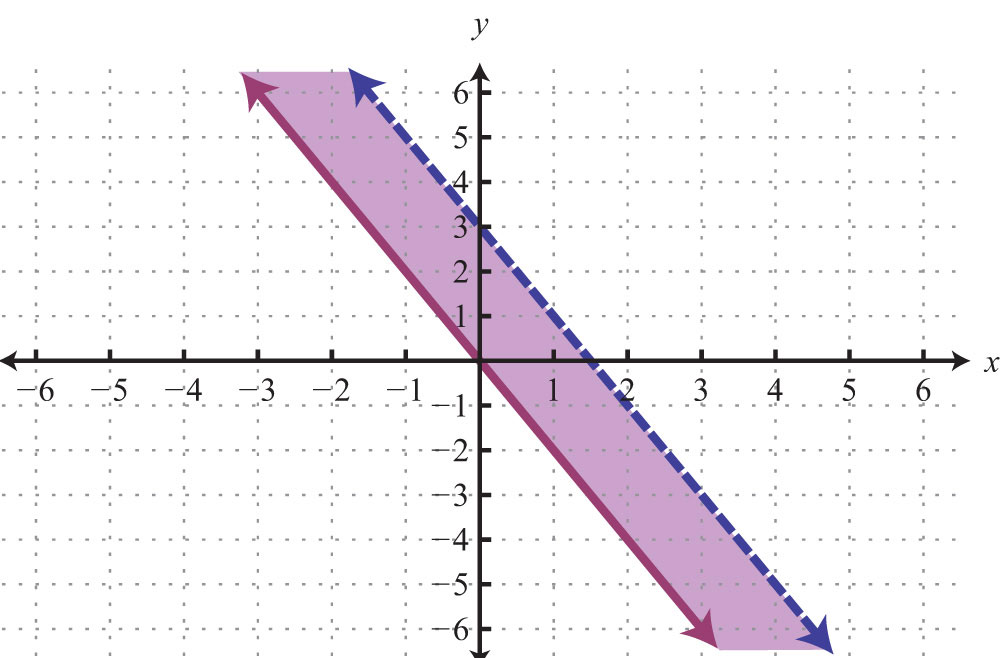31: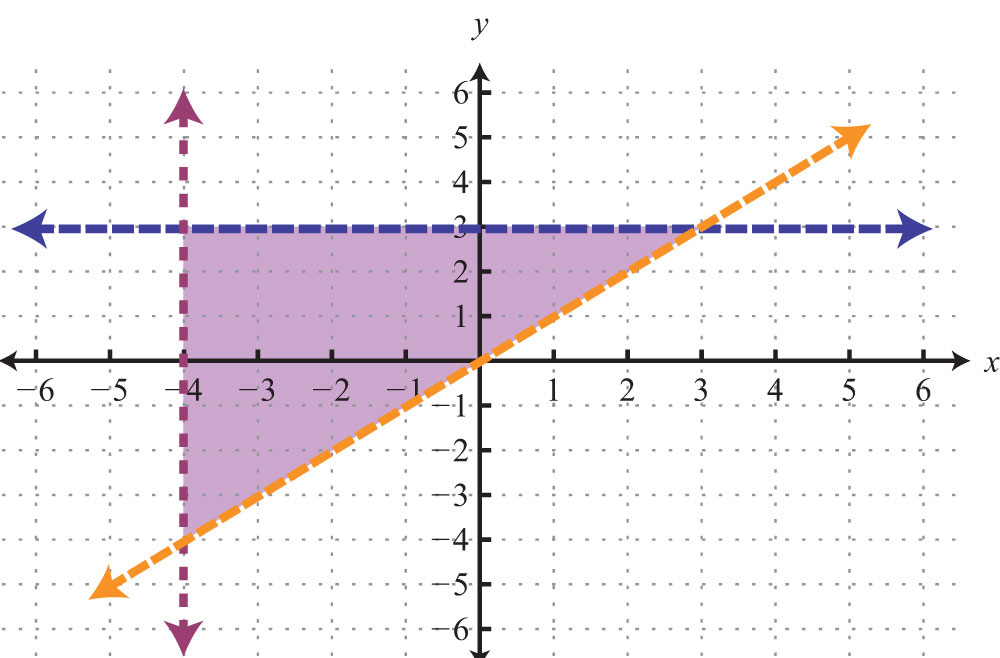33: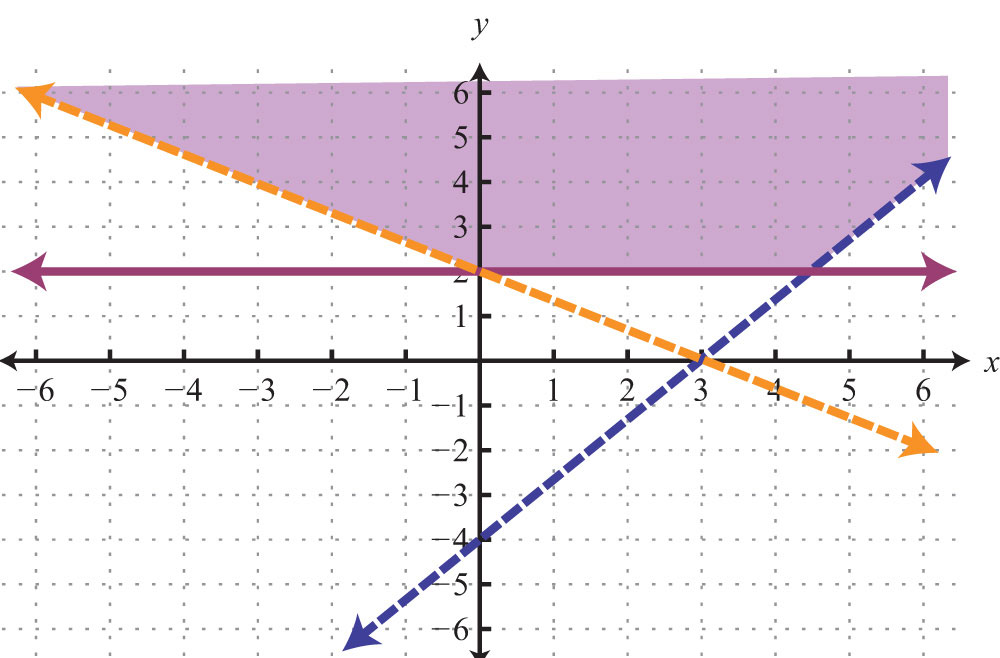35: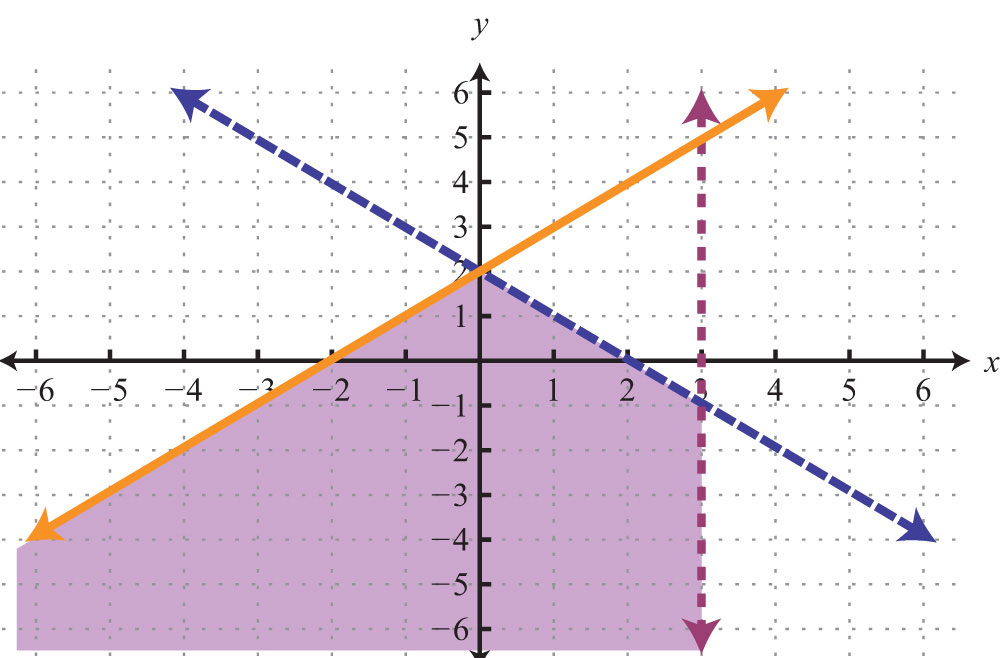37: ${x>0y>0$

39: ${x<0y<0$